Home » Wiki » Variable frequency drive linear V/F characteristic

# Variable frequency drive linear V/F characteristic

The variable frequency drive sensorless control in configuration is based on the proportional change of output voltage compared to the output frequency according to the VFD configured characteristic.

By setting the variable frequency drive V/f-characteristic, the voltage of the connected 3-phase motor is controlled according to the frequency. The torque to be applied by the motor at the corresponding operating point demands the control of the output voltage proportional to the frequency. At a constant output voltage/output frequency ratio of the variable frequency drive, the magnetization is constant in the nominal operating range of the 3-phase motor. The rating point of the motor or end point of the V/f-characteristic is set via the guided commissioning with the parameter Cut-Off Voltage and the parameter Cut-Off Frequency.

The lower frequency range, where an increased voltage is necessary for the start of the VFD, is critical. The voltage at an output frequency of zero is set with parameter Starting Voltage. A voltage increase deviating from the linear course of the V/f-characteristic can be defined by parameters Voltage Rise and Rise Frequency. The VFD parameter value percentage is calculated from the linear V/f-characteristic. Via the parameters Minimum Frequency and Maximum Frequency, the working range of the motor or the V/f-characteristic is defined.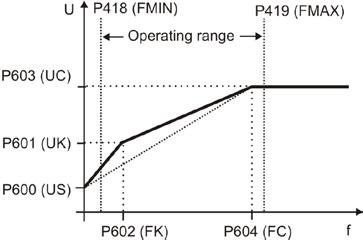(FMIN): Minimum Frequency, (FMAX): Maximum Frequency,
(US): Starting Voltage,
(UK): Voltage Rise, (FK): Rise Frequency
(UC): Cut-Off Voltage, (FC): Cut-Off Frequency

Note:
The guided commissioning takes the parameterized rated motor values and reference data of the variable frequency drive into account when it comes to presetting the V/f-characteristic. In the case of asynchronous machines, the speed can be increased at a constant torque if the motor winding can be switched over from star to delta connection. If the data for delta connection indicated on the rating plate of the asynchronous motor were entered, the cut-off frequency is increased automatically by the square root of three.

The Cut-Off Voltage (UC) and Cut-Off Frequency (FC) set in the factory are derived from the motor data Rated Voltage and Rated Frequency. With the parameterized Starting Voltage (US), the linear equation of the variable frequency drive V/f-characteristic results.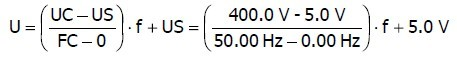The Rise Frequency (FK) is entered as a percentage of the Cut-Off Frequency (FC), the default value is f = 10 Hz. The output voltage for the default Voltage Rise is calculated as U = 92.4 V.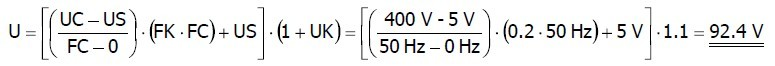Plz Calculate (1 * 9) =
(Your comment will show after approved.)

You may also like:

Category
Featured Articles
Variable frequency drive Rectifier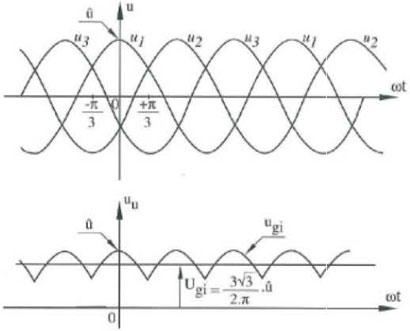To understand variable frequency drive (VFD) better, it's necessary to explain some of the main parts of the variable frequency ...
VFD controlled Induction motor ...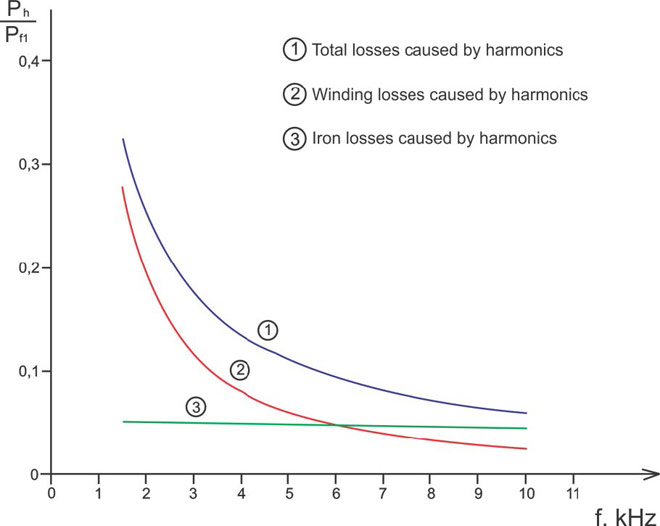This paper presents a procedure to measure the efficiency on an induction motor fed by a VFD by the all operation range to speed ...
VFD: Pulse Width Modulation (PWM)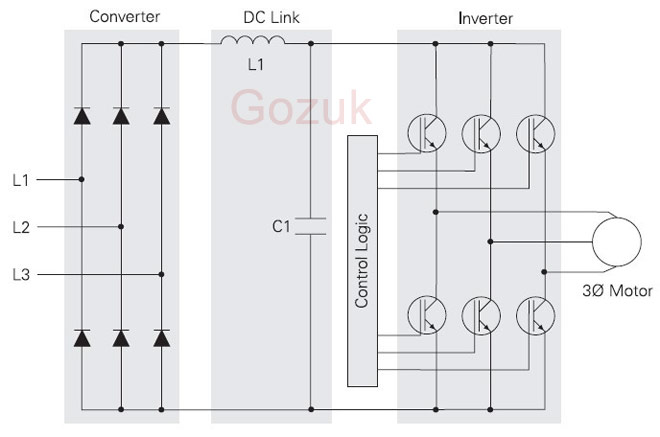Pulse Width Modulation (PWM) VFDs provide a more sinusoidal current output to control frequency and voltage supplied to an AC ...
Variable frequency drive Advantages & ... VFDs are good for variable speed, in a water pump this is used to maintain a steady pressure, they will smooth out variances in ...
Variable frequency drive Energy saving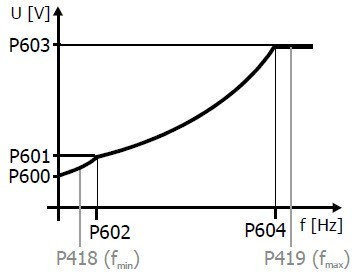Energy can be saved in a VFD by reducing the losses in the electric motor or by reducing the energy consumption of the variable ...VFD manufacturers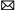buy@vfds.orgtech@vfds.org